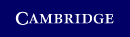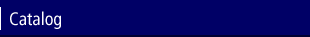Home > Catalog > Optimization Methods in FinanceSearch this bookDetails

• 43 b/w illus. 44 tables 177 exercises
• Page extent: 358 pages
• Size: 247 x 174 mm
• Weight: 0.772 kg

Library of Congress

• Dewey number: 332.015196
• Dewey version: 22
• LC Classification: n/a
• Finance--Mathematical models
• Mathematical optimization

Library of Congress Record

Hardback

(ISBN-13: 9780521861700)

Replaced by 9781107056749

\$89.99 (P)

Optimization models play an increasingly important role in financial decisions. This is the first textbook devoted to explaining how recent advances in optimization models, methods and software can be applied to solve problems in computational finance more efficiently and accurately. Chapters discussing the theory and efficient solution methods for all major classes of optimization problems alternate with chapters illustrating their use in modeling problems of mathematical finance. The reader is guided through topics such as volatility estimation, portfolio optimization problems and constructing an index fund, using techniques such as nonlinear optimization models, quadratic programming formulations and integer programming models respectively. The book is based on Master's courses in financial engineering and comes with worked examples, exercises and case studies. It will be welcomed by applied mathematicians, operational researchers and others who work in mathematical and computational finance and who are seeking a text for self-learning or for use with courses.

Contents

1. Introduction; 2. Linear programming: theory and algorithms; 3. LP models: asset/liability cash flow matching; 4. LP models: asset pricing and arbitrage; 5. Nonlinear programming: theory and algorithms; 6. NLP volatility estimation; 7. Quadratic programming: theory and algorithms; 8. QP models: portfolio optimization; 9. Conic optimization tools; 10. Conic optimization models in finance; 11. Integer programming: theory and algorithms; 12. IP models: constructing an index fund; 13. Dynamic programming methods; 14. DP models: option pricing; 15. DP models: structuring asset backed securities; 16. Stochastic programming: theory and algorithms; 17. SP models: value-at-risk; 18. SP models: asset/liability management; 19. Robust optimization: theory and tools; 20. Robust optimization models in finance; Appendix A. Convexity; Appendix B. Cones; Appendix C. A probability primer; Appendix D. The revised simplex method; Bibliography; Index.

Reviews

"This book will be useful as a textbook for students in financial engineering at the MS level.... The book will also be of interest to researchers and graduate students in optimization who are interested in applications of optimization to financial problems."
Brian Borchers, Journal of Online Mathematics and its Applications

'This book would certainly appeal to someone with a mathematical background, perhaps in operations research, wishing to update and apply their knowledge to the financial world.' Mathematics TODAY

'Until now, there has been no comprehensive optimization book aimed at quantitative analysts in the financial industry. The book by Cornuejols and Tutuncu fills this void … an excellent source for quantitative financial analysts and graduate students to learn about basic optimization theory, computational methods, and available software. At the same time, it can be used by academic researchers and students in optimization as an introduction to various interesting problems in financial applications.' International Review of Economics & Finance